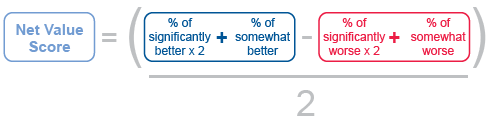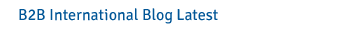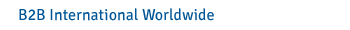Calculating The Net Value Score

Using the data from the question on total value, carry out the following steps:

1. Double the percentage of people that stated “significantly better”.
2. Double the percentage of people that stated “significantly worse”.
3. Add the adjusted “significantly better” figure (from step 1) to the percentage of people that stated “somewhat better”.
4. Add the adjusted “significantly worse” figure (from step 2) to the percentage of people that stated “somewhat worse”.
5. Subtract the total “worse” calculation (from step 4) from the total “better” calculation (from step 3).
6. Half the score to arrive at the Net Value Score.
Calculating The Net Value ScoreAn example of a response table and the calculation of the Net Value Score is as follows:

Percentage giving a response Calculation of the
Net Value Score
Significantly better 33% +66
Somewhat better 20% +20
Neither better nor worse 30% 0
Somewhat worse 10% -10
Significantly worse 7% -14
TOTAL 100% Equation: (86 - 24) / 2
Net Value Score = +31

In the example above, the company has achieved a Net Value Score of +31 out of a maximum score of 100. This should then be compared to the Net Value Score of each competitor in order to understand the relative perceived value of each player in the market.

The Net Value Score is a composite measure of perceived brand value. The maximum possible score is 100. Benchmarks are as follows:

• Outstanding: 60+
• Excellent: 40-59
• Good: 30-39
• Average: 20-29
• Poor: <20RSS Feed Widget